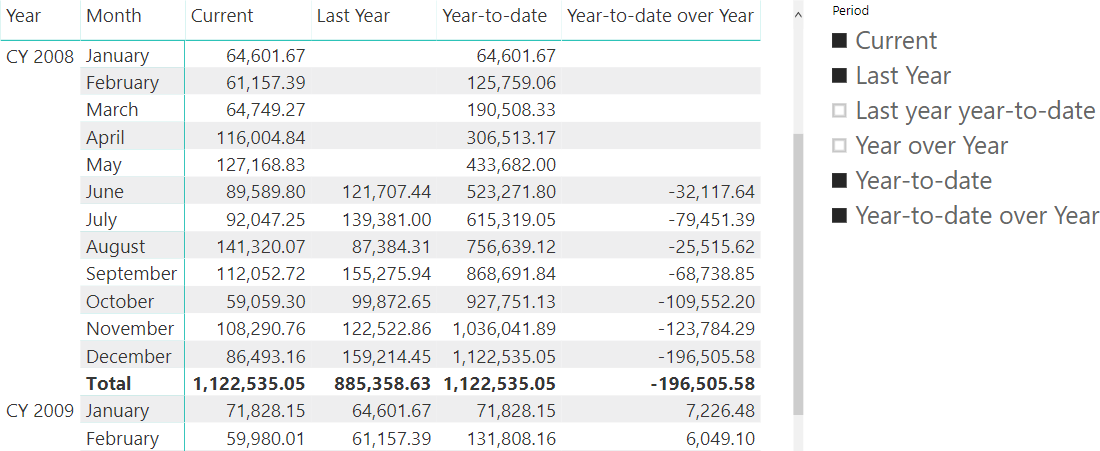In DAX, variables are useful to write more readable code. Variables are also useful to optimize code execution, because a good usage of variables prevents multiple evaluations of the same expression. However, in certain conditions their use could be counterproductive, negatively affecting performance.

First, let us demonstrate how variables can improve code execution. For example, consider the following report computing the year-to-date over last year’s year-to-date, only considering months where there are sales in both years.The measure computing the last column of the report is the following one.

```Sales YTDOY :=
CALCULATE (
SUMX (
VALUES ( 'Date'[Calendar Year Month Number] ),
VAR CurrentSales = [Sales Amount]
VAR PreviousSales = [Sales LY]
RETURN
IF (
AND (
CurrentSales <> 0,
PreviousSales <> 0
),
CurrentSales - PreviousSales
)
),
DATESYTD ( 'Date'[Date] )
)
```

The Sales YTDOY measure evaluates Sales Amount and Sales LY only once for each month, using the value in the IF statement and in the following subtraction. This is good, because the alternative is a shorter piece of code invoking the same measures multiple times.

```Sales YTDOY slow :=
CALCULATE (
SUMX (
VALUES ( 'Date'[Calendar Year Month Number] ),
IF (
AND (
[Sales Amount] <> 0,
[Sales LY] <> 0
),
[Sales Amount] - [Sales LY]
)
),
DATESYTD ( 'Date'[Date] )
)
```

Though the DAX engine might reuse the result obtained for the same measures in the same filter context (Sales Amount and Sales LY), this is not always the case. In this scenario, variables are a good way to ensure a better optimized code execution.

However, variables should only be used within their respective scope. For example, if a variable is defined before a conditional statement, then the variable will be evaluated regardless of the condition. This has a strong performance impact in case there are disconnected slicers in the report. To elaborate on this, consider the following report where a Time Selection table is used to define a slicer that controls which columns of the matrix should be visible. The matrix contains a single measure called Sales, whose content depends on the period selected in the column.The Sales measure using the variables can be defined this way:

```Sales vSlow =
VAR PeriodSelection =
SELECTEDVALUE ( 'Time Selection'[PeriodSelector] )
VAR SalesAmount = [Sales Amount]
VAR SalesLY = [Sales LY]
VAR SalesYOY = [Sales YOY]
VAR SalesYTD = [Sales YTD]
VAR SalesLYTD = [Sales LYTD]
VAR SalesYTDOY = [Sales YTDOY]
RETURN
SWITCH (
PeriodSelection,
"C", SalesAmount,
"LY", SalesLY,
"YOY", SalesYOY,
"YTD", SalesYTD,
"LYTD", SalesLYTD,
"YTDOY", SalesYTDOY,
BLANK ()
)
```

There are no specific reasons to write the code this way for this measure, because each variable is only used once. However, one might reuse the variables in multiple expressions of PeriodSelection, reducing the measures defined in the data model. Nevertheless, we might expect to obtain the same performance for the following code:

```Sales vFast =
VAR PeriodSelection =
SELECTEDVALUE ( 'Time Selection'[PeriodSelector] )
RETURN
SWITCH (
PeriodSelection,
"C", [Sales Amount],
"LY", [Sales LY],
"YOY", [Sales YOY],
"YTD", [Sales YTD],
"LYTD", [Sales LYTD],
"YTDOY", [Sales YTDOY],
BLANK ()
)
```

After all, we are evaluating the same conditional statement; we expect the variables to be evaluated only when they are used later in the DAX expression. However, an optimization is available for the IF and SWITCH functions, called short-circuit evaluation. It is implemented by applying a particular filter to the filter context of the branches of the IF and SWITCH evaluation. Because the variables in the Sales vSlow measure are defined in a different scope before IF and SWITCH, the change of filter context does not apply to the variable evaluation. Therefore, all the variables (and all the measures) are evaluated regardless of the selection made in the Period slicer of the sample report.

The consequence of this behavior can severely affect the performance of a well-structured set of DAX measures. Therefore, it is better to follow these guidelines when using variables:

• When the same DAX expression is evaluated multiple times within the same filter context, assign it to a variable and reference the variable instead of the DAX expression.
• When a DAX expression is evaluated within the branches of IF or SWITCH, whenever necessary assign the expression to a variable within the conditional branch – this will maintain the short-circuit evaluation optimization.
• Do not assign a variable outside an IF or SWITCH statement if the variable is used only within the conditional branch.
• The first argument of IF and SWITCH can consume variables defined before IF and SWITCH without it affecting performance.
IFChecks whether a condition is met, and returns one value if TRUE, and another value if FALSE.

`IF ( <LogicalTest>, <ResultIfTrue> [, <ResultIfFalse>] )`

SWITCHReturns different results depending on the value of an expression.

`SWITCH ( <Expression>, <Value>, <Result> [, <Value>, <Result> [, … ] ] [, <Else>] )`